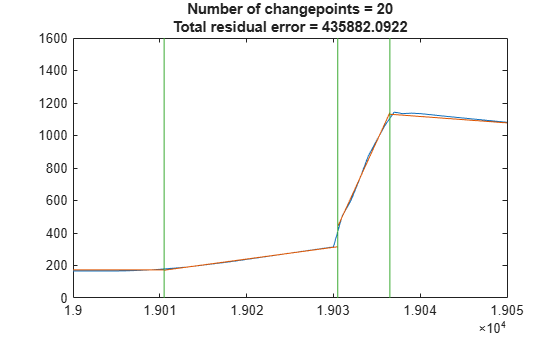# 检测信号的爆发和重大变化

### 通过累积和检测爆发

```load WestAfricanEbolaOutbreak2014 plot(WHOreportdate, [TotalCasesGuinea TotalCasesLiberia TotalCasesSierraLeone],'.-') legend('Guinea','Liberia','Sierra Leone') title('Total Suspected, Probable, and Confirmed Cases of Ebola Virus Disease')```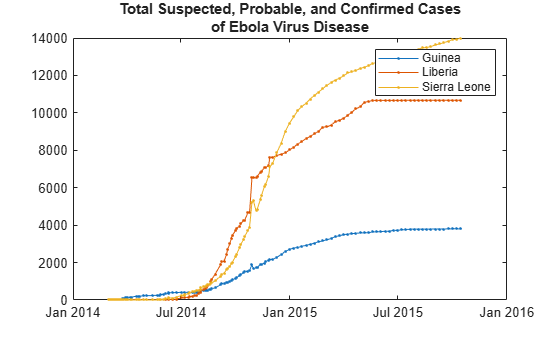```daysSinceOutbreak = datetime(2014, 3, 24+(0:400)); cases = interp1(WHOreportdate, TotalCasesLiberia, daysSinceOutbreak); dayOverDayCases = diff(cases); plot(dayOverDayCases) title('Rate of New Cases (per Day) in Liberia since March 25, 2014'); ylabel('Change in Number of Reported Cases per Day'); xlabel('Number of Days from Start of Outbreak');```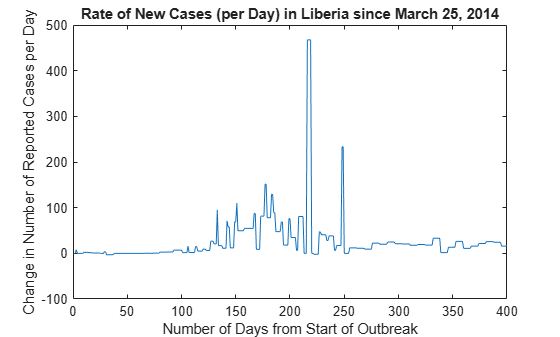`xlim([1 101])`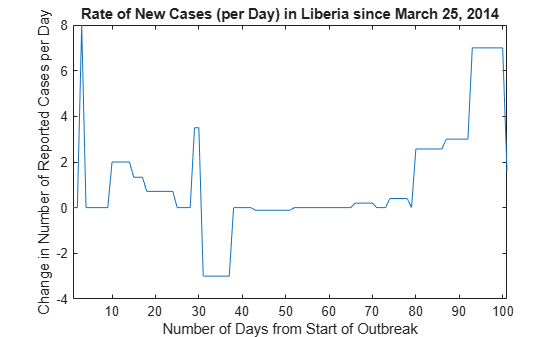```cusum(dayOverDayCases(1:101)) legend('Upper sum','Lower sum')```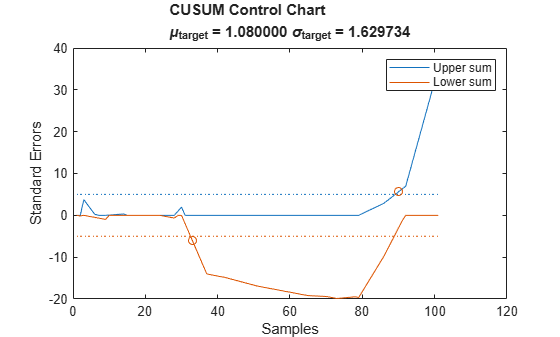```climit = 5; mshift = 1; tmean = 0; tdev = 3; cusum(dayOverDayCases(1:100),climit,mshift,tmean,tdev)```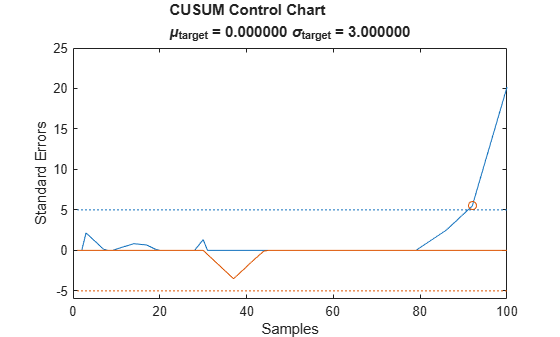### 发现方差的显著变化

```load nilometer years = 622:1284; plot(years,nileriverminima) title('Yearly Minimum level of Nile River') xlabel('Year') ylabel('Level (m)')```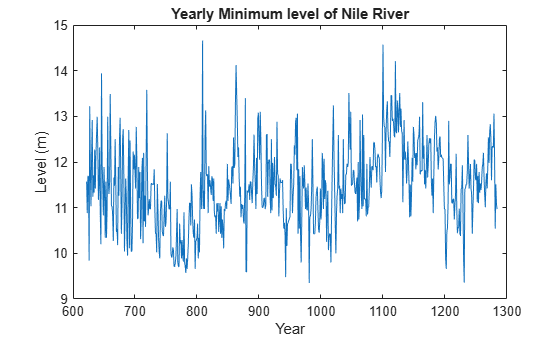```i = findchangepts(diff(nileriverminima),'Statistic','rms'); ax = gca; xp = [years(i) ax.XLim([2 2]) years(i)]; yp = ax.YLim([1 1 2 2]); patch(xp,yp,[.5 .5 .5],'FaceAlpha',0.1)```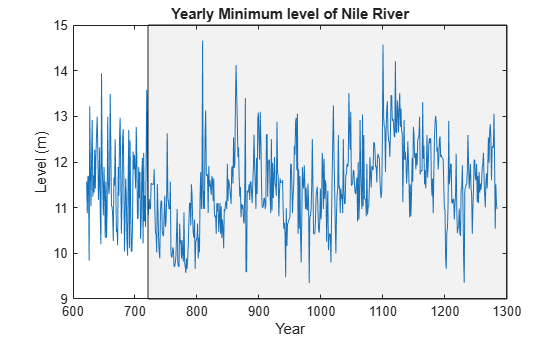### 检测输入信号的多个变化

```load simcarsig subplot(2,1,2) plot(carTorqueNM) xlabel('Samples') ylabel('Torque (N m)') title('Torque') subplot(2,1,1); plot(carEngineRPM) xlabel('Samples') ylabel('Speed (RPM)') title('Engine Speed')```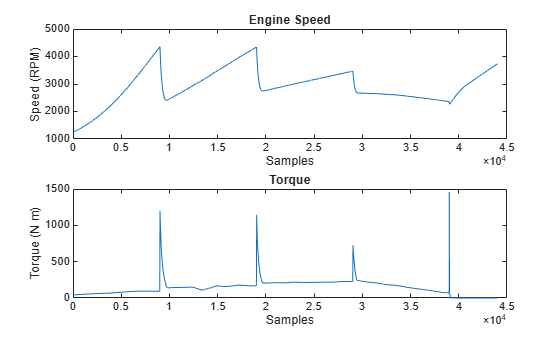```figure findchangepts(carEngineRPM,'Statistic','linear','MaxNumChanges',4) xlabel('Samples') ylabel('Engine speed (RPM)')```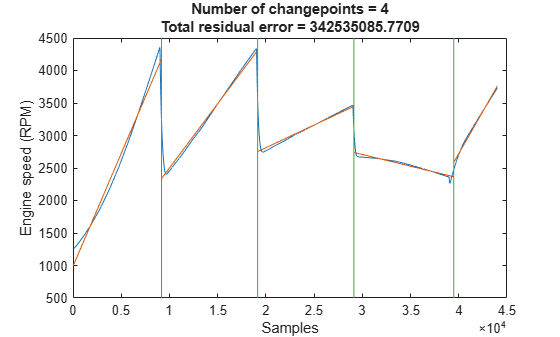`xlim([18000 22000])`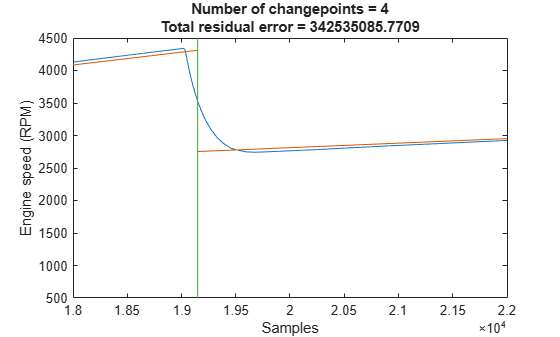### 观察信号间共享的多阶段事件的变化

```findchangepts(carEngineRPM,'Statistic','linear','MaxNumChanges',20) xlim([18000 22000])```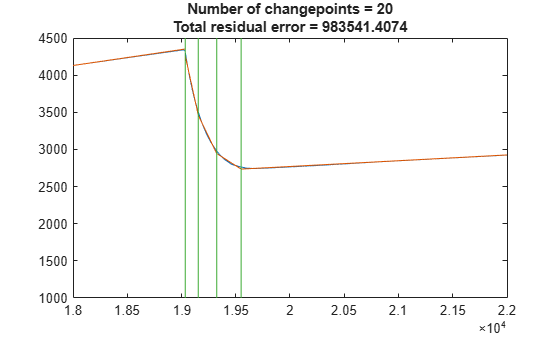```findchangepts(carTorqueNM,'Statistic','Linear','MaxNumChanges',20) xlim([19000 20000])```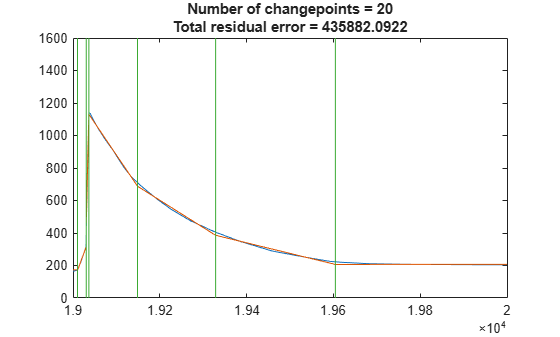`xlim([19000 19050])`